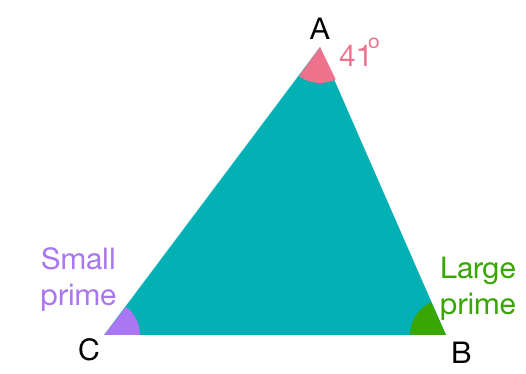# Prime AnglesAngle $A$ is 41 degrees, angle $B$ is larger than angle $C,$ and all three angles are prime numbers.

What is the measure of angle $B$?

Clarification: All the angles are measured in degrees.

×